# Lossless Compression Techniques

January 30, 2021

By  Prithvi

lang: en_US

## Data Compression

Data compression is a reduction in the number of bits needed to represent data. Compressing data can save storage capacity, speed up file transfer, and decrease costs for storage hardware and network bandwidth. Lossless compression techniques are  a class of data compression algorithms that allows the original data to be perfectly reconstructed from the compressed data. By contrast, lossy compression permits reconstruction only of an approximation of the original data, though usually with greatly improved compression rates (and therefore reduced media sizes).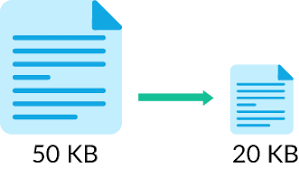## Huffman Coding

A Huffman code is a particular type of optimal prefix code that is commonly used for lossless data compression. This means that the codes (bit sequences) are assigned in such a way that the code assigned to one character is not the prefix of code assigned to any other character. The output from Huffman's algorithm can be viewed as a variable-length code table for encoding a source symbol (such as a character in a file). As in other entropy encoding methods, more common symbols are generally represented using fewer bits than less common symbols.

The technique works by creating a binary tree of nodes. These can be stored in a regular array, the size of which depends on the number of symbols ‘n’. Initially, all nodes are leaf nodes, which contain the symbol itself, the weight (frequency of appearance) of the symbol, and optionally, a link to a parent node which makes it easy to read the code (in reverse) starting from a leaf node. Internal nodes contain a weight(probability), links to two child nodes, and an optional link to a parent node. In our implementation, the weight is considered in terms of probability and the parent node is the composite sum of the child node probabilities.

Huffman coding occurs in stages which can be defined by the

number of stages,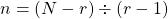N=Number of symbols,

r=number of digits used to represent 1 number of the code.

In our case, N=4,r=2 so 'n' is =2, which is the number of stages.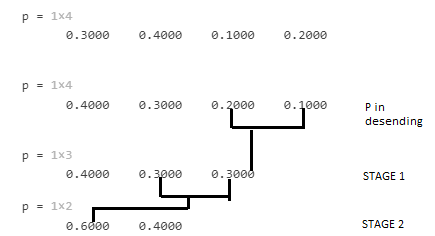• CODE

• output

%%%%%%%%%%%%%%%%%%%%%%%%%%%%%%%%%%%%%%%%%%
%% Topic:Lossless compression techniques using MATLAB
%Author:Prithvi Kishan
%Implementing Huffman encoding on numeric symbols using MATLAB functions.
clear all;
close all;
clc;
%% Generating numbers from 1 to 8 as symbols
symbols = 1:8;
%Each symbol has its repective probablity defined by p
prob = [.5 .125 .125 .125 .03125 .03125 .03125 .03125 ];
%Generate a binary Huffman code dictionary
hdict = huffmandict(symbols,prob);
%Generating a 100x1 inputsignal with values from 1 to 8
inputSig = randsrc(100,1,[symbols;prob]);
%% Converting the original signal to a binary, and determining the length of the binary symbols.
binarySig = de2bi(inputSig);
ip_seq_len_binary = numel(binarySig)
%% Generating a lossless, compressed ecoded signal
code = huffmanenco(inputSig,hdict);
%Converting the Huffman-encoded symbols to binary, and determine the length of the encoded binary symbols.
binaryComp = de2bi(code);
enc_seq_len_binary = numel(binaryComp)
%% Decoding the Huffman encoded code and comapring with original input
sig = huffmandeco(code,hdict);
if(isequal(inputSig,sig))
display('Huffman decoded signal and original signal are equal hence it is lossless');
end

## Shannon’s binary encoding theorem

Shannon coding is a lossless data compression technique for constructing a prefix code based on a set of symbols and their probabilities (estimated or measured). It is suboptimal in the sense that it does not achieve the lowest possible expected codeword length as Huffman coding does, and never better but sometimes equal to the Shannon–Fano coding.

The technique used is, the symbols are arranged in descending order of probability, and assigned codewords by taking the first integer greater than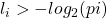(done using ceil function in MATLAB) bits from the binary expansions of the cumulative probabilities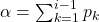which are initially multiplied by 2 and rounded off to the smallest integer lass than it( done using floor function in MATLAB).

• CODE

• INPUT

• output

%%%%%%%%%%%%%%%%%%%%%%%%%%%%%%%%%%%%%%%%
% Topic:Lossless compression techniques using MATLAB
%Author:Prithvi Kishan
%Implementing Shannon binary encoding.
clc;
clear all;
close all;
%% Taking in symbols and their probabilities
m=input('Enter the number  of message symbols : ');
code=[];
h=0;l=0;
display('Enter the probabilities in descending order');
for i=1:m
fprintf('Symbol %d probability \n',i);
p(i)=input('');
end
%% Finding alpha and code length array
%Finding each symbols' alpha values by adding probablities of previous symbol
a(1)=0;
for j=2:m;
a(j)=a(j-1)+p(j-1);
end
fprintf('\n Alpha Matrix');
display(a);
%Finding each code length
for i=1:m
n(i)= ceil(-1*(log2(p(i))));
end
fprintf('\n Code length matrix');
display(n);
%% Implementing shannons binary encoding algoritm
for i=1:m
int=a(i);
for j=1:n(i)
frac=int*2;
c=floor(frac);
frac=frac-c;
code=[code c];
int=frac;
end
fprintf('Codeword %d',i);
display(code);
code=[];
end
%% Computing Important compression algorithm parameters
%Computing average code length
fprintf('Avgerage Code Length');
for i=1:m
x=p(i)*n(i);
l=l+x;
x=p(i)*log2(1/p(i));
h=h+x;
end
display(l);
%Computing Entropy, effieciency and redundancy
fprintf('Entropy');
display(h);
fprintf('Efficiency');
display(100*h/l);
fprintf('Redundancy');
display(100-(100*h/l));

## Conclusion

Huffman coding and Shannon’s binary encoding techniques offer good information compression which is essential in this era of vast streams of information exchange to help in bandwidth conservation and to improve speeds. Shannon’s binary encoding although helps in lossless compression it had an efficiency of ~80% whereas Huffman coding has more than 95% in most scenarios and hence is not the optimal compression algorithm. Newer algorithms like LZ77, LZ78, LZR, etc have been developed that surpasses the efficiency of the above-mentioned algorithms.

Ready to take your MATLAB skills to the next level? Look no further! At MATLAB Helper, we've got you covered. From free community support to expert help and training, we've got all the resources you need to become a pro in no time. If you have any questions or queries, don't hesitate to reach out to us. Simply post a comment below or send us an email at [email protected].

And don't forget to connect with us on LinkedIn, Facebook, and Subscribe to our YouTube Channel! We're always sharing helpful tips and updates, so you can stay up-to-date on everything related to MATLAB. Plus, if you spot any bugs or errors on our website, just let us know and we'll make sure to fix it ASAP.

Ready to get started? Book your expert help with Research Assistance plan today and get personalized assistance tailored to your needs. Or, if you're looking for more comprehensive training, join one of our training modules and get hands-on experience with the latest techniques and technologies. The choice is yours – start learning and growing with MATLAB Helper today!

Education is our future. MATLAB is our feature. Happy MATLABing!

#### Prithvi

Engineering student with a keen interest to learn and implement new things. Currently working as a MATLAB developer at MATLAB helper.

#### MATLAB Helper ®

Use Website Chat or WhatsApp at +91-8104622179